## RS Aggarwal Class 9 Solutions Chapter 2 Polynomials Ex 2D

These Solutions are part of RS Aggarwal Solutions Class 9. Here we have given RS Aggarwal Solutions Class 9 Chapter 2 Polynomials Ex 2D.

Other Exercises

Using factor theorem, show that :

Question 1.
Solution:
By factor theorem, x – 2 will be a factor of f(x) = x3 – 8 if f(2) = 0
(∴ x-2 = 0=>x = 2)
Now f(2) = (2)3 – 8 = 8- 8 = 0
Hence (x – 2) is a factor of f(x) Ans.

Question 2.
Solution:
By factor theorem,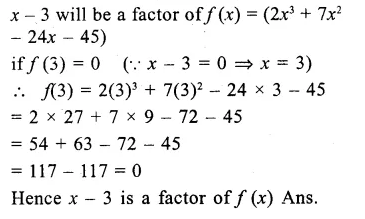Question 3.
Solution:
By factor theorem,
(x – 1) is a factor of f(x)=(2x4 + 9x3 + 6x2– 11x – 6)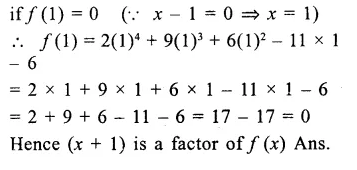Question 4.
Solution:
By factor theorem, (x + 2) will
a factor of f (x) = x4 – x4 + 2Question 5.
Solution:
By factor theorem, (x + 5) will be a factor of f(x) = 2x3 + 9x2 – 11x – 30 if f(-5) = 0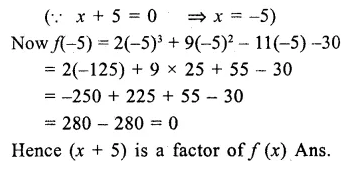Question 6.
Solution:
By factor theorem, (2x – 3) is a factor of f(x) = 2x4 + x3 – 8x2 – x + 6Question 7.
Solution:
By factor theorem, (x – √2 ) will be a factor of f(x) = 7x2 – 4√2x – 6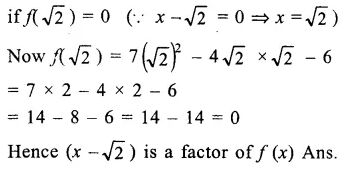Question 8.
Solution:
By factor theorem, (x + √2) will be a factor of f(x) = 2√2 x3 + 5x + √2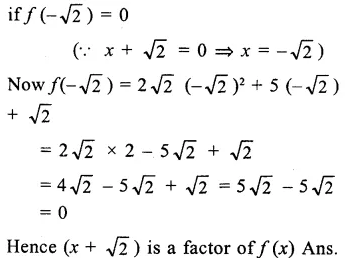Question 9.
Solution:
Let f(x) = 2x3 + 9x2 + x + k and x – 1 is a factor of f(x)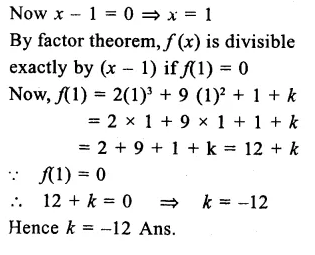Question 10.
Solution:
Let f(x) = 2x3 – 3x2 – 18x + a and x – 4 is its factorQuestion 11.
Solution:
Let f(x) – x4 – x3 – 11x2 – x + a
f(x) is divisible by (x + 3)Question 12.
Solution:
Let f(x) = 2x3 + ax2 + 11x + a + 3
and (2x – 1) is its factor
Let 2x – 1 = 0 then 2x = 1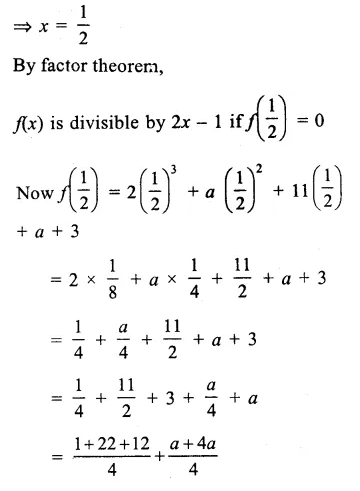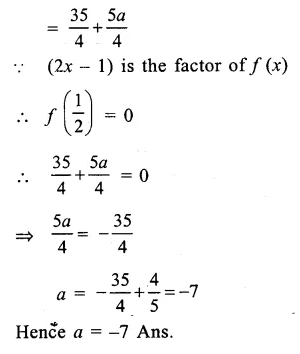Question 13.
Solution:
Let f(x) = x3 – 10x2 + ax + b and (x – 1) and (x – 2) are its factors
∴ x – 1 = 0 =>x=1
and x – 2 = 2 =>x=2Question 14.
Solution:
Let f(x) = x4 + ax3 – 7x2 – 8x + b ,
and (x + 2) and (x + 3) are its factors
∴x + 2 = 0 => x = -2
and x + 3= 0 => x = -3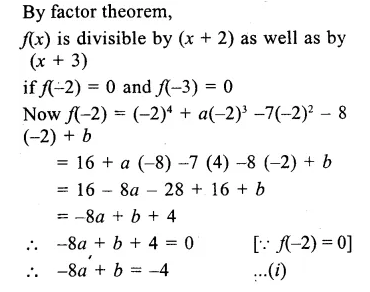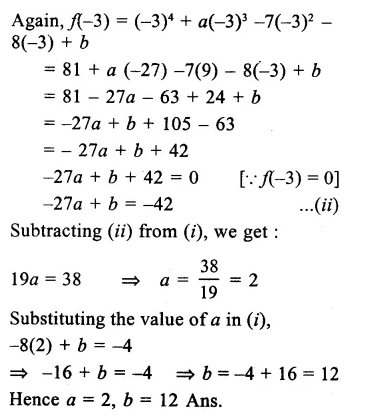Question 15.
Solution:
Let f(x) = x3 – 3x2 – 13x + 15
Now x2 + 2x – 3 = x2 + 3x – x – 3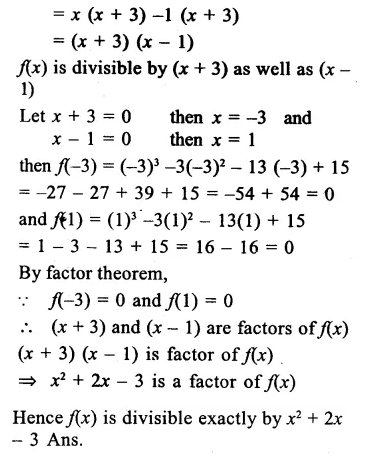Question 16.
Solution:
Let f(x) = x3 + ax2 + bx + 6 and (x – 2) is its factor
Let x – 2 = 0 then x = 2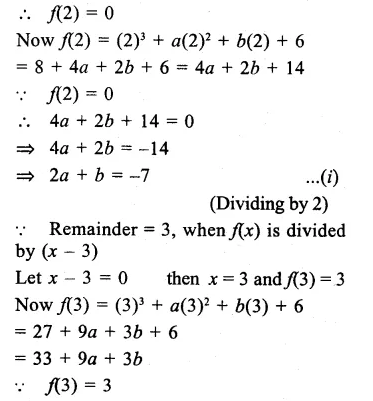Hope given RS Aggarwal Solutions Class 9 Chapter 2 Polynomials Ex 2D are helpful to complete your math homework.

If you have any doubts, please comment below. Learn Insta try to provide online math tutoring for you.